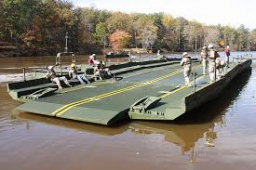# Bridge diagonally across the river

The width of the river is 89 m. For terrain reasons, the bridge deviates from a common perpendicular to both banks by an angle of 12° 30 '. Calculate how many meters the bridge is longer than the river.

x =  2.1609 m

### Step-by-step explanation:Did you find an error or inaccuracy? Feel free to write us. Thank you!Tips to related online calculators
Do you want to convert length units?

#### You need to know the following knowledge to solve this word math problem:

We encourage you to watch this tutorial video on this math problem:

## Related math problems and questions:

• Balloon and bridgeFrom the balloon, which is 92 m above the bridge, one end of the bridge is seen at a depth angle of 37° and the second end at depth angle 30° 30 '. Calculate the length of the bridge.
• RiverFrom the observatory 11 m high and 24 m from the riverbank, river width appears in the visual angle φ = 13°. Calculate the width of the river.
• 30-60-90The longer leg of a 30°-60°-90° triangle measures 5. What is the length of the shorter leg?
• DitchDitch profile is an isosceles trapezoid with bases of length 80m and 60m. The slope of the side wall of the ditch is 80°. Calculate the ditch depth.
• TrianglesFind out whether given sizes of the angles can be interior angles of a triangle: a) 23°10',84°30',72°20' b) 90°,41°33',48°37' c) 14°51',90°,75°49' d) 58°58',59°59',60°3'
• Right triangle trigonometricsCalculate the size of the remaining sides and angles of a right triangle ABC if it is given: b = 10 cm; c = 20 cm; angle alpha = 60° and the angle beta = 30° (use the Pythagorean theorem and functions sine, cosine, tangent, cotangent)
• Inner anglesThe inner angles of the triangle are 30°, 45° and 105° and its longest side is 10 cm. Calculate the length of the shortest side, write the result in cm up to two decimal places.
• AircraftFrom the aircraft flying at an altitude of 500m, they observed places A and B (located at the same altitude) in the direction of flight at depth angles alpha = 48° and beta = 35°. What is the distance between places A and B?
• Water channelThe cross section of the water channel is a trapezoid. The width of the bottom is 19.7 m, the water surface width is 28.5 m, the side walls have a slope of 67°30' and 61°15'. Calculate how much water flows through the channel in 5 minutes if the water flo
• ReflectorCircular reflector throws light cone with a vertex angle 49° and is on 33 m height tower. The axis of the light beam has with the axis of the tower angle 30°. What is the maximum length of the illuminated horizontal plane?
• MastMast has 13 m long shadow on a slope rising from the mast foot in the direction of the shadow angle at angle 13.3°. Determine the height of the mast, if the sun above the horizon is at angle 45°12'.
• BuildingThe building I focused at an angle 30°. When I moved 5 m building I focused at an angle 45°. What is the height of the building?
• Resultant forceCalculate mathematically and graphically the resultant of three forces with a common center if: F1 = 50 kN α1 = 30° F2 = 40 kN α2 = 45° F3 = 40 kN α3 = 25°
• Two boatsTwo boats are located from a height of 150m above the surface of the lake at depth angles of 57° and 39°. Find the distance of both boats if the sighting device and both ships are in a plane perpendicular to the surface of the lake.
• BuildingHow high is the building that throws horizontal shadow 85.6 m long at angle 34°12'?
• Right angleIn a right triangle ABC with a right angle at the apex C, we know the side length AB = 24 cm and the angle at the vertex B = 71°. Calculate the length of the legs of the triangle.
• A trapezoidA trapezoid with a base length of a = 36.6 cm, with angles α = 60°, β = 48° and the height of the trapezoid is 20 cm. Calculate the lengths of the other sides of the trapezoid.